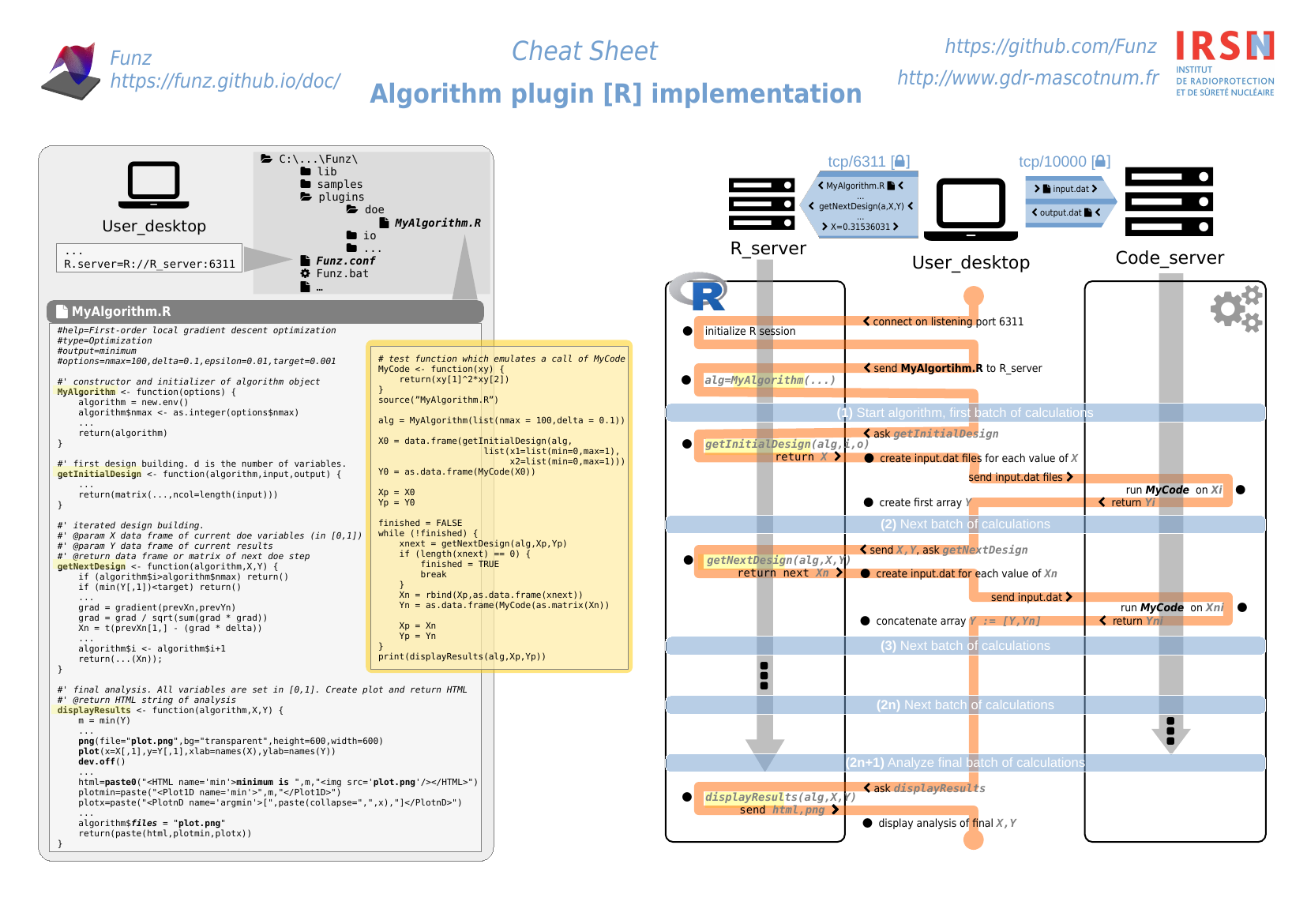# Design algorithm

## Overview

Funz design ‘algorithm’ is the component that features funz to drive an external simulation software for an engineering objective. It mainly provides some methods in R language to define numerical simulations to perform, and returns information about engineering target.For now, following algorithms are available out-of-the-box:

For conveniency, a basic template is provided and should be used as a scratch project.

## Installation

Once installed, Funz can integrate some more algorithms. Python and R wrappers provide `install.Design('ALGORITHM')` methods to add one of the previously bundled.

From scratch (when no bundle is available), you can add a new algorithm by hand just by copying file ‘MyAlgorithm.R’ inside ‘Funz/plugins/doe/’ directory.

## Bundle implementation

### Step-by-step

These steps will guide you to build a basic algorithm, which is sufficient for most of your needs.

1. Checkout the algorithm template:
2. Edit the ‘build.xml’ file to replace `MyAlgorithm` by the name of your algorithm (usually, the name of the simulation software)
3. Edit the ‘README.md’ description file
4. Rename and implement the ‘src/main/doe/MyAlgorithm.R’ file:
`````` #title:...
#help:...
#author:...
#type:...
#output:...
#options:...
#options.help:...
``````
• fill the constructor:
`````` #' constructor and initializer of algorithm
#' @param options algorithm options
#' @return algorithm object : environment options, status
MyAlgorithm <- function(options) {
...
algorithm = new.env()
...
return(algorithm)
}
``````
• fill the initalizer of experiments (typically with random points):
`````` #' first design building.
#' @param algorithm object handling options, status, ...
#' @param input the input variables and their properties (like min, max)
#' @param output the output targets names
#' @return matrix of first design step
getInitialDesign <- function(algorithm,input,output) {
...
return(matrix(...,ncol=length(input)))
}
``````
• fill the iterator (considering previous calculations resutls):
`````` #' iterated design building.
#' @param algorithm object handling options, status, ...
#' @param X matrix of current doe variables
#' @param Y matrix of current results
#' @return matrix of next doe step
getNextDesign <- function(algorithm,X,Y) {
...
return(matrix(...,ncol=ncol(X)))
}
``````
• fill the results renderer (with plots & string return):
`````` #' final analysis.
#' @param algorithm object handling options, status, ...
#' @param X matrix of current doe variables
#' @param Y matrix of current results
#' @return HTML string of analysis
displayResults <- function(algorithm,X,Y) {
...
return(html)
}
``````
5. Provide (at least) one test case ‘src/test/cases/MyTest.R’ containing R::testthat tests:
`````` ## This file should provide following objects, when loaded:
# f : function
# input.f : list of input dimensions, contains list of properties like lower & upper bounds of each dimensions
# output.f : list of output dimensions
# *.f : list of math properties. To be compared with algorithm results
# [print.f] : method to print/plot the function for information

f = function(X) { return(cos(pi*x)) }

input.f = list(
x=list(min=0,max=1)
)
output.f = "cos_pi"
root.f = 0.5

test = function(algorithm_file) {
results = run.algorithm(algorithm_file, options=NULL,fun=list(input=input.f,output=output.f,fun=f))
library(testthat)
test_that("cos_pi info",{expect_equal(as.numeric(results\$info),root.f,tolerance = .0001)})
}
``````
6. Check all test cases using `ant test`, which returns a json report, and should not finished by `FAILED`